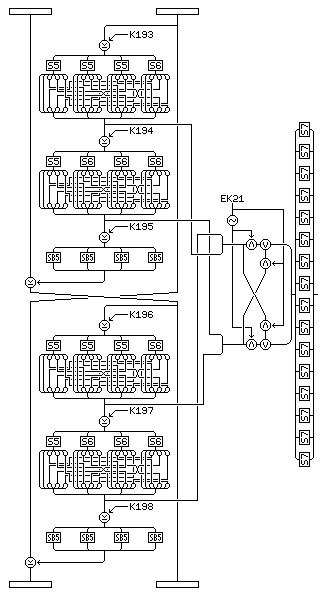[Next] [Up] [Previous] [Index]

# Quadibloc 2002E: Introduction to the Core Rounds; Left Block Half

The eleven standard rounds subject the block to a very strong diffusion, and, which, with the bit swaps, also create a certain level of algorithmic variability. In Quadibloc 2002E, however, they are supplemented with rounds of a different type. These rounds, in two groups of four, constitute the "cryptographic core" of the cipher, to use a term introduced with the MARS block cipher, despite the fact that the eleven rounds defined so far certainly are strong in their own right.

After each of the first three rounds in a group of four of these rounds, the halves of the block are swapped.

Thus, the overall structure of Quadibloc 2002E is:

```Four standard rounds
Four core rounds
Three standard rounds
Four core rounds
Four standard rounds
```

with the standard rounds as described on the previous page.

Four rounds of Quadibloc 2002D, or four rounds of Quadibloc 2002, with suitable S-box replacements, would serve to produce a strong cipher in these positions. The round actually proposed for this purpose has some similarities to the Quadibloc 2002D round. The operation performed on the left half of the block is almost identical, except for the key-dependent S-box being designated SB5 instead of S7, and except for changing the direction of the f-function and introducing a swap halves operation in order to allow the cipher, with modifications to the key schedule, to invert itself:but the remaining operations are different, although the overall structure of the round is similar. Also, note the bit swap applied to the intermediate result output; this is also done to permit the cipher to act as its own inverse.

The operation performed on the left half of the block consists of two Feistel rounds, with a swap of 32-bit halves of this half in between, which are similar to the operation used for Quadibloc S. In each case, the right half of the 64-bit subblock is used as the input to the f-function, and the left-half of the subblock is modified, since the reverse is the case in the combiner acting on the other half of the 128-bit block, thus requiring only an exchange of 64-bit halves of the block between cryptographic core rounds to maintain alternation of f-function input halves and modified halves.

The sequence of operations within the f-function is as follows:

• The input into the f-function is XORed with the first of the three 32-bit subkeys used for the f-function.
• The result is divided into four bytes. These bytes are then replaced by their substitutes found in S-boxes S5, S5, S5, and S6 respectively, where S5 and S6 are the fifth and sixth constant S-boxes derived from Euler's constant as described on the page concerning Euler's Constant and the Quadibloc S-boxes.
• The bits of the result, considered to be numbered from 1 (most significant bit of the first, leftmost byte) to 32 (least significant bit of the last, rightmost byte) following the pattern in DES, are to be transposed to lie in the following positions:
``` 1  2 27 28 21 22 15 16
9 10  3  4 29 30 23 24
17 18 11 12  5  6 31 32
25 26 19 20 13 14  7  8
```
• The current value of the result is the first intermediate result of this f-function.
• The result is XORed with the second of the three 32-bit subkeys used for the f-function.
• The result is divided into four bytes. These bytes are then replaced by their substitutes found in S-boxes S5, S6, S6, and S6 respectively, with S5 and S6 as described above.
• The bits of the result are transposed according to the transposition previously described.
• The current value of the result is the second intermediate result of this f-function.
• The result is XORed with the third subkey used for this f-function.
• The result is divided into four bytes, each of which is then replaced by its substitute found in the key-dependent S-box SB5.
• The current value is the output from the f-function.

Thus, the left half of the 128-bit block is modified as follows: its left half is XORed with the f-function of the right half, then its two 64-bit halves are swapped, and again the left half is XORed with the f-function of the right half.

The four intermediate results produced by this are arranged as follows, from left to right, to produce a 128-bit value:

```the first intermediate result of the second f-function
the second intermediate result of the first f-function
the first intermediate result of the first f-function
the second intermediate result of the second f-function
```

Next, shown and described here, is the first part of the overall f-function for a cryptographic core round in Quadibloc 2002E:

Then, a 64-bit exchange key is applied to this 128-bit value, being considered as divided into two 64-bit halves; where a bit in the exchange key is a zero, corresponding bits in the two halves are unaffected; where a bit in the exchange key is a one, corresponding bits in the two halves are switched.

Then, the 128-bit value is considered as being composed of sixteen bytes, and each byte is replaced by its substitute in S-box S7, again as described in the section on Euler's Constant and the Quadibloc S-boxes.

The 128-bit output from these manipulations now is modified by means of the main part of the main f-function for the round, to be described on the next page.

[Next] [Up] [Previous] [Index]